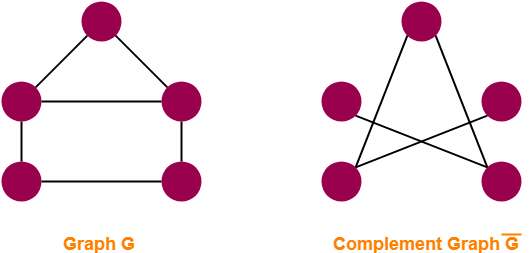# Complement of Graph in Graph Theory | Example | Problems

## Complement Of Graph-

Complement of a simple graph G is a simple graph G’ having-

• All the vertices of G.
• An edge between two vertices v and w iff there exists no edge between v and w in the original graph G.

## Complement Of Graph Example-

The following example shows a graph G and its complement graph G’-## Relationship Between G & G’-

The following relationship exists between a graph G and its complement graph G’-

1. Number of vertices in G = Number of vertices in G’.

 |V(G)| = |V(G’)|

2. The sum of total number of edges in G and G’ is equal to the total number of edges in a complete graph.

 |E(G)| + |E(G’)| = C(n,2) = n(n-1) / 2

where n = total number of vertices in the graph

## Important Terms-

It is important to note the following terms-

• Order of graph = Total number of vertices in the graph
• Size of graph = Total number of edges in the graph

Also Read- Types of Graphs in Graph Theory

## Problem-01:

A simple graph G has 10 vertices and 21 edges. Find total number of edges in its complement graph G’.

## Solution-

Given-

• Number of edges in graph G, |E(G)| = 21
• Number of vertices in graph G, n = 10

We know |E(G)| + |E(G’)| = n(n-1) / 2.

Substituting the values, we get-

21 + |E(G’)| = 10 x (10-1) / 2

|E(G’)| = 45 – 21

∴ |E(G’)| = 24

Thus, Number of edges in complement graph G’ = 24.

## Problem-02:

A simple graph G has 30 edges and its complement graph G’ has 36 edges. Find number of vertices in G.

## Solution-

Given-

• Number of edges in graph G, |E(G)| = 30
• Number of edges in graph G’, |E(G’)| = 36

We know |E(G)| + |E(G’)| = n(n-1) / 2.

Substituting the values, we get-

30 + 36 = n(n-1) / 2

n(n-1) = 132

n2 – n – 132 = 0

Solving this quadratic equation, we get n = 12.

Thus, Number of vertices in graph G = 12.

## Problem-03:

Let G be a simple graph of order n. If the size of G is 56 and the size of G’ is 80. What is n?

## Solution-

Size of a graph = Number of edges in the graph.

Given-

• Number of edges in graph G, |E(G)| = 56
• Number of edges in graph G’, |E(G’)| = 80

We know |E(G)| + |E(G’)| = n(n-1) / 2.

Substituting the values, we get-

56 + 80 = n(n-1) / 2

n(n-1) = 272

n2 – n – 272 = 0

Solving this quadratic equation, we get n = 17.

Thus, Number of vertices in graph G = 17.

In other words, Order of graph G = 17.

To gain better understanding about Complement Of Graph,

Watch this Video Lecture

Next Article- Walks in Graph Theory

Get more notes and other study material of Graph Theory.

Watch video lectures by visiting our YouTube channel LearnVidFun.

SummaryArticle Name
Complement of Graph in Graph Theory | Example | Problems
Description
Complement of Graph in Graph Theory- Complement of a graph G is a graph G' with all the vertices of G in which there is an edge between two vertices v and w if and only if there exist no edge between v and w in the original graph G. Complement of Graph Examples and Problems.
Author
Publisher Name
Gate Vidyalay
Publisher Logo